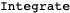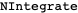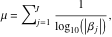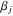# Volume 23

## Selected Financial Applications »NBPDF

Published December 16, 2021

This article shows how to use some of Mathematicas built-in financial functions and define new functions useful for the practical analysis of real-world financial data. The main topics covered are linear programming and its application in bond portfolio management, conditional value-at-risk minimization, introductory time-series analysis, simulation, bootstrapping, robust equity portfolio optimization and artificial intelligence. Read More »

## Mixing Numbers and Unfriendly Colorings of Graphs »NBPDF

Published September 28, 2021

Mathematica has many built-in functions for doing research in graph theory. Formerly it was necessary to load the Combinatorica package to access these functions; most are now available within Mathematica itself. This article studies a problem concerning the vertex coloring of graphs using Mathematica by introducing some user-defined functions. Read More »

## Numerical Contour Integration »NBPDF

### Applied to One- and Two-Dimensional Integrals over Distributions

Published July 16, 2021

We present a straightforward implementation of contour integration by setting options forand, taking advantage of powerful results in complex analysis. As such, this article can be viewed as documentation to perform numerical contour integration with the existing built-in tools. We provide examples of how this method can be used when integrating analytically and numerically some commonly used distributions, such as Wightman functions in quantum field theory. We also provide an approximating technique when time-ordering is involved, a commonly encountered scenario in quantum field theory for computing second-order terms in Dyson series expansion and Feynman propagators. We believe our implementation will be useful for more general calculations involving advanced or retarded Greens functions, propagators, kernels and so on. Read More »

## Unconditional Applicability of Lehmer’s Measure to the Two-Term Machin-like Formula for π »NBPDF

Published May 24, 2021

Lehmer defined a measurewhere themay be either integers or rational numbers in a Machin-like formula for. When theare integers, Lehmers measure can be used to determine the computational efficiency of the given Machin-like formula for. However, because the computations are complicated, it is unclear if Lehmers measure applies when one or more of theare rational. In this article, we develop a new algorithm for a two-term Machin-like formula foras an example of the unconditional applicability of Lehmers measure. This approach does not involve any irrational numbers and may allow calculatingrapidly by the NewtonRaphson iteration method for the tangent function. Read More »

## Coverage versus Confidence »NBPDF

### A Tutorial

Published March 3, 2021

This article is intended to help students understand the concept of a coverage probability involving confidence intervals. Mathematica is used as a language for describing an algorithm to compute the coverage probability for a simple confidence interval based on the binomial distribution. Then, higher-level functions are used to compute probabilities of expressions in order to obtain coverage probabilities. Several examples are presented: two confidence intervals for a population proportion based on the binomial distribution, an asymptotic confidence interval for the mean of the Poisson distribution, and an asymptotic confidence interval for a population proportion based on the negative binomial distribution. Read More »